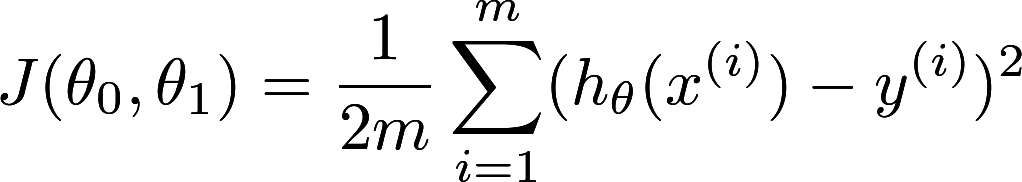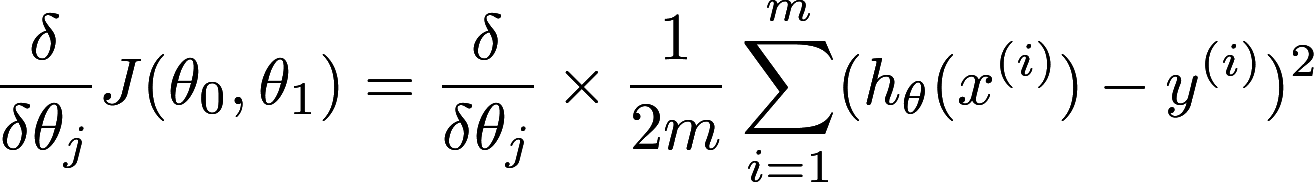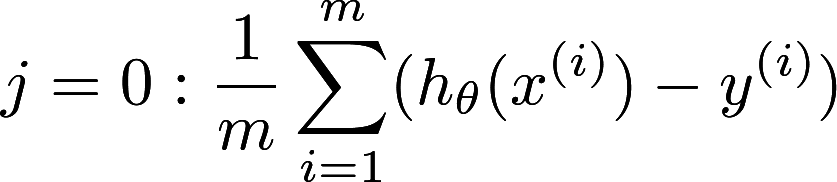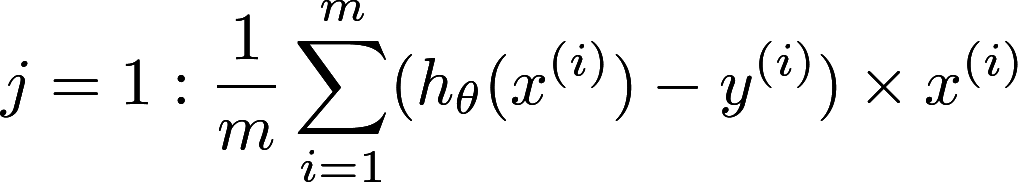Why Machine learning tools need GPU to shine — a deeper look from software perspective
Post
Cancel

# Why Machine learning tools need GPU to shine — a deeper look from software perspective

In our previous article, we have discovered GPU programming in the most unexpected place - an API of a SaaS product. It is the JQL of Mixpanel. And in this article we will explore further about the relationship of GPU programming and machine learning or deep learning. They are the most trendy terms in the tech world nowadays. So what is the connection between machine learning / deep learning tools and GPU programming? I guess every software developers will immediately scream “parallelization” and call it a day. But why is that so? Why is parallelization has anything to do with machine learning and GPU programming?

Let’s take the easiest machine learning algorithm as an example. The algorithm is regression. Regression is the easiest algorithm to understand. It is one of the algorithm that is taught in secondary school, back in the days when I was studying. What it does is to find the most descriptive equation to describe all the data points in the dataset. How? By giving trying different equation and give a point to the equation. Whichever equation that scores the lowest is the most suitable equation for the data points. And to simplify the illustration further, we take the univariate version of linear regression, which basically means there is only 1 parameter to predict another parameter. Those parameters for example, could be using one’s height to predict one’s weight.

The algorithm can be split into two parts: the cost function “J(θ)” and its derivative J`(θ).The cost function is also called “mean squared error” function. Mean squared function is very literal. It gives a score of the hypothesis function. The score is based on the mean of the squared difference between the predicted result(x) and actual result(y). So in the equation, we can already relate to the previous post about reducer. See the summation sign(∑)? The summation sign is a typical pattern for reduction.

How about the derivative of cost function? What is the use of the function? Differentiation comes into handy in here. The function is to find out the “slope” of the cost function. If the cost function is minimal, the “slope” of the cost function will tends to zero. The actual linear algebra is explained perfectly in Mrs Chow’s class when I was in F5. But it is not available online. So we must use Andrew Ng’s course as a substitute.

Disregarding the underlying explanation, just take a look as the equation itself. See the summation sign(∑) again? Yes. The summation sign is a pattern of reduction as mentioned before. The derivative of cost function can be efficiently solved by GPU. Therefore both component of linear regression can be solved efficiently by GPU! Voilà!

So here is the problem for the next article: we can take two paths, we can either discuss the different patterns on parallelization, or we can discuss what other machine learning algorithms which can make use of parallelization? The former one is more software engineering and the later one is more machine learning. Let me know what you think!